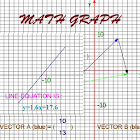Math Graph

All Android applications categories

All Android games categories# Math Graph

by: 192 5.8

5.8 Users
rating

## Screenshots

Description

This app will help beginners in graphical methods understand the linear equation, y=mx+c. Learn about linear graphs and vectors simply by just a swipe. Select graphs or vectors on intro page.

Find the relationship between lines, gradients, y-intercept, linear equations and vectors by drawing a graph with your finger while this app does the rest.

No input of values is necessary. This is an automatic graph app.

Math Graph app – you draw graph, it works out the equation for you.

Trace the graph with your finger. When done, sit back and study your graph.

The app will draw the line and calculate gradient.

It will also write down the graph equation at bottom left of screen.

On vectors screen, you draw vectors with your finger, and this app analyzes them for you. It is an easy way to learn about simple vectors including addition of vectors.

This native math app is meant for beginners in graphical methods, the first 2 years of learning graphs.

If you have studied complex graphical methods but would still like to remind yourself about the basics, this app will do just that.

You can work with the equations produced at your leisure, re-arranging them to discover more about graphical mathematics.

Tags: maths work out down , math graph , draw graph using equation , graphe maths , math app graph , maths graphe

from 192 reviews

"Good"

5.8